# DeMorgan's Theorem

A mathematician named DeMorgan developed a pair ofimportant rules regarding group complementation in Boolean algebra. By group complementation, I’m referring to the complement of a group of terms, represented by a long bar over more than one variable.

You should recall from the chapter on logic gates that inverting all inputs to a gate reverses that gate’s essential function from AND to OR, or vice versa, and also inverts the output. So, an OR gate with all inputs inverted (a Negative-OR gate) behaves the same as a NAND gate, and an AND gate with all inputs inverted (a Negative-AND gate) behaves the same as a NOR gate. DeMorgan’s theorems state the same equivalence in “backward” form: that inverting the output of any gate results in the same function as the opposite type of gate (AND vs. OR) with inverted inputs: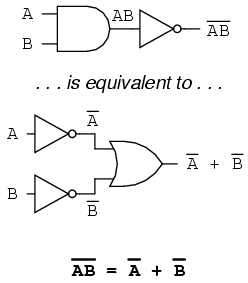A long bar extending over the term AB acts as a grouping symbol, and as such is entirely different from the product of A and B independently inverted. In other words, (AB)’ is not equal to A’B’. Because the “prime” symbol (‘) cannot be stretched over two variables like a bar can, we are forced to use parentheses to make it apply to the whole term AB in the previous sentence. A bar, however, acts as its own grouping symbol when stretched over more than one variable. This has profound impact on how Boolean expressions are evaluated and reduced, as we shall see.

DeMorgan’s theorem may be thought of in terms of breaking a long bar symbol. When a long bar is broken, the operation directly underneath the break changes from addition to multiplication, or vice versa, and the broken bar pieces remain over the individual variables. To illustrate: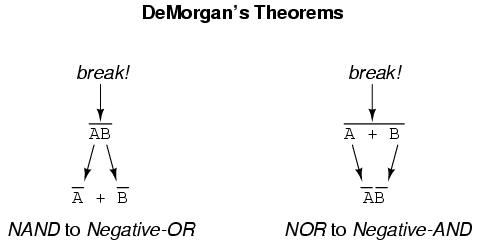When multiple “layers” of bars exist in an expression, you may only break one bar at a time, and it is generally easier to begin simplification by breaking the longest (uppermost) bar first. To illustrate, let’s take the expression (A + (BC)’)’ and reduce it using DeMorgan’s Theorems:Following the advice of breaking the longest (uppermost) bar first, I’ll begin by breaking the bar covering the entire expression as a first step: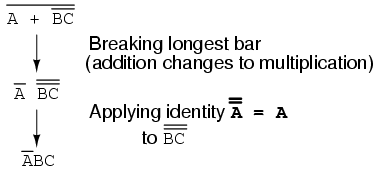As a result, the original circuit is reduced to a three-input AND gate with the A input inverted:You should never break more than one bar in a single step, as illustrated here:As tempting as it may be to conserve steps and break more than one bar at a time, it often leads to an incorrect result, so don’t do it!

It is possible to properly reduce this expression by breaking the short bar first, rather than the long bar first:The end result is the same, but more steps are required compared to using the first method, where the longest bar was broken first. Note how in the third step we broke the long bar in two places. This is a legitimate mathematical operation, and not the same as breaking two bars in one step! The prohibition against breaking more than one bar in one step is not a prohibition against breaking a bar in more than one place. Breaking in more than one place in a single step is okay; breaking more than one bar in a single step is not.

You might be wondering why parentheses were placed around the sub-expression B’ + C’, considering the fact that I just removed them in the next step. I did this to emphasize an important but easily neglected aspect of DeMorgan’s theorem. Since a long bar functions as a grouping symbol, the variables formerly grouped by a broken bar must remain grouped lest proper precedence (order of operation) be lost. In this example, it really wouldn’t matter if I forgot to put parentheses in after breaking the short bar, but in other cases it might. Consider this example, starting with a different expression:As you can see, maintaining the grouping implied by the complementation bars for this expression is crucial to obtaining the correct answer.

Let’s apply the principles of DeMorgan’s theorems to the simplification of a gate circuit:As always, our first step in simplifying this circuit must be to generate an equivalent Boolean expression. We can do this by placing a sub-expression label at the output of each gate, as the inputs become known. Here’s the first step in this process:Next, we can label the outputs of the first NOR gate and the NAND gate. When dealing with inverted-output gates, I find it easier to write an expression for the gate’s output without the final inversion, with an arrow pointing to just before the inversion bubble. Then, at the wire leading out of the gate (after the bubble), I write the full, complemented expression. This helps ensure I don’t forget a complementing bar in the sub-expression, by forcing myself to split the expression-writing task into two steps:Finally, we write an expression (or pair of expressions) for the last NOR gate:Now, we reduce this expression using the identities, properties, rules, and theorems (DeMorgan’s) of Boolean algebra: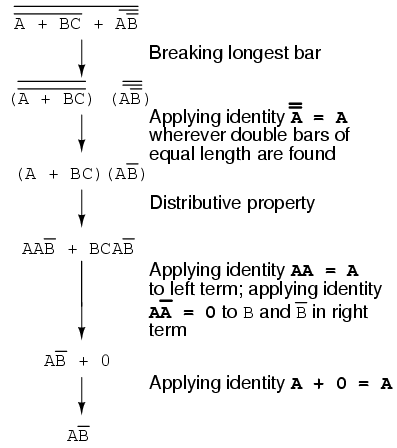The equivalent gate circuit for this much-simplified expression is as follows: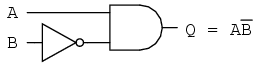• REVIEW
• DeMorgan’s Theorems describe the equivalence between gates with inverted inputs and gates with inverted outputs. Simply put, a NAND gate is equivalent to a Negative-OR gate, and a NOR gate is equivalent to a Negative-AND gate.
• When “breaking” a complementation bar in a Boolean expression, the operation directly underneath the break (addition or multiplication) reverses, and the broken bar pieces remain over the respective terms.
• It is often easier to approach a problem by breaking the longest (uppermost) bar before breaking any bars under it. You must never attempt to break two bars in one step!
• Complementation bars function as grouping symbols. Therefore, when a bar is broken, the terms underneath it must remain grouped. Parentheses may be placed around these grouped terms as a help to avoid changing precedence.

## Converting truth tables into Boolean expressions

In designing digital circuits, the designer often begins with a truth table describing what the circuit should do. The design task is largely to determine what type of circuit will perform the function described in the truth table. While some people seem to have a natural ability to look at a truth table and immediately envision the necessary logic gate or relay logic circuitry for the task, there are procedural techniques available for the rest of us. Here, Boolean algebra proves its utility in a most dramatic way.

To illustrate this procedural method, we should begin with a realistic design problem. Suppose we were given the task of designing a flame detection circuit for a toxic waste incinerator. The intense heat of the fire is intended to neutralize the toxicity of the waste introduced into the incinerator. Such combustion-based techniques are commonly used to neutralize medical waste, which may be infected with deadly viruses or bacteria:So long as a flame is maintained in the incinerator, it is safe to inject waste into it to be neutralized. If the flame were to be extinguished, however, it would be unsafe to continue to inject waste into the combustion chamber, as it would exit the exhaust un-neutralized, and pose a health threat to anyone in close proximity to the exhaust. What we need in this system is a sure way of detecting the presence of a flame, and permitting waste to be injected only if a flme is “proven” by the flame detection system.

Several different flame-detection technologies exist: optical (detection of light), thermal (detection of high temperature), and electrical conduction (detection of ionized particles in the flame path), each one with its unique advantages and disadvantages. Suppose that due to the high degree of hazard involved with potentially passing un-neutralized waste out the exhaust of this incinerator, it is decided that the flame detection system be made redundant (multiple sensors), so that failure of a single sensor does not lead to an emission of toxins out the exhaust. Each sensor comes equipped with a normally-open contact (open if no flame, closed if flame detected) which we will use to activate the inputs of a logic system: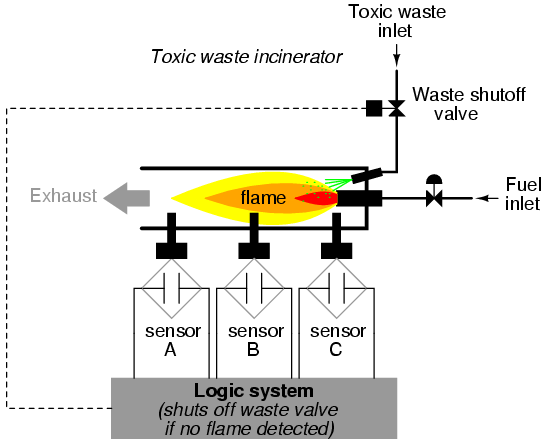Our task, now, is to design the circuitry of the logic system to open the waste valve if and only if there is good flame proven by the sensors. First, though, we must decide what the logical behavior of this control system should be. Do we want the valve to be opened if only one out of the three sensors detects flame? Probably not, because this would defeat the purpose of having multiple sensors. If any one of the sensors were to fail in such a way as to falsely indicate the presence of flame when there was none, a logic system based on the principle of “any one out of three sensors showing flame” would give the same output that a single-sensor system would with the same failure. A far better solution would be to design the system so that the valve is commanded to open if any only if all three sensors detect a good flame. This way, any single, failed sensor falsely showing flame could not keep the valve in the open position; rather, it would require all three sensors to be failed in the same manner — a highly improbable scenario — for this dangerous condition to occur.

Thus, our truth table would look like this: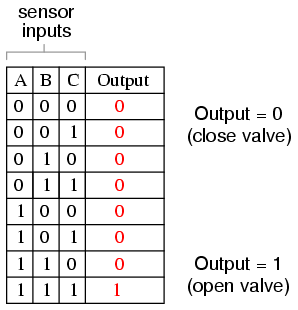It does not require much insight to realize that this functionality could be generated with a three-input AND gate: the output of the circuit will be “high” if and only if input A AND input B AND input C are all “high:”If using relay circuitry, we could create this AND function by wiring three relay contacts in series, or simply by wiring the three sensor contacts in series, so that the only way electrical power could be sent to open the waste valve is if all three sensors indicate flame: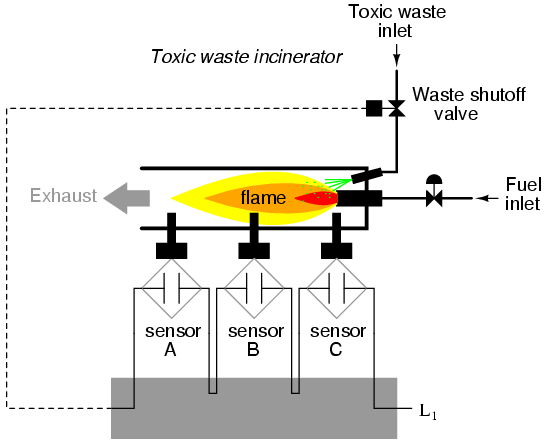While this design strategy maximizes safety, it makes the system very susceptible to sensor failures of the opposite kind. Suppose that one of the three sensors were to fail in such a way that it indicated no flame when there really was a good flame in the incinerator’s combustion chamber. That single failure would shut off the waste valve unnecessarily, resulting in lost production time and wasted fuel (feeding a fire that wasn’t being used to incinerate waste).

It would be nice to have a logic system that allowed for this kind of failure without shutting the system down unnecessarily, yet still provide sensor redundancy so as to maintain safety in the event that any single sensor failed “high” (showing flame at all times, whether or not there was one to detect). A strategy that would meet both needs would be a “two out of three” sensor logic, whereby the waste valve is opened if at least two out of the three sensors show good flame. The truth table for such a system would look like this:Here, it is not necessarily obvious what kind of logic circuit would satisfy the truth table. However, a simple method for designing such a circuit is found in a standard form of Boolean expression called the Sum-Of-Products, or SOP, form. As you might suspect, a Sum-Of-Products Boolean expression is literally a set of Boolean terms added (summed) together, each term bein a multiplicative (product) combination of Boolean variables. An example of an SOP expression would be something like this: ABC + BC + DF, the sum of products “ABC,” “BC,” and “DF.”

Sum-Of-Products expressions are easy to generate from truth tables. All we have to do is examine the truth table for any rows where the output is “high” (1), and write a Boolean product term that would equal a value of 1 given those input conditions. For instance, in the fourth row down in the truth table for our two-out-of-three logic system, where A=0, B=1, and C=1, the product term would be A’BC, since that term would have a value of 1 if and only if A=0, B=1, and C=1:Three other rows of the truth table have an output value of 1, so those rows also need Boolean product expressions to represent them:Finally, we join these four Boolean product expressions together by addition, to create a single Boolean expression describing the truth table as a whole: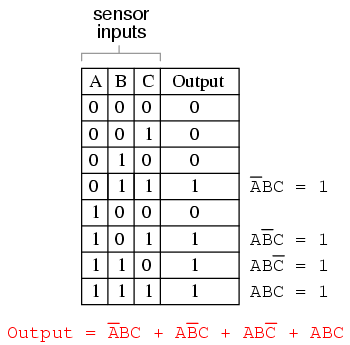Now that we have a Boolean Sum-Of-Products expression for the truth table’s function, we can easily design a logic gate or relay logic circuit based on that expression: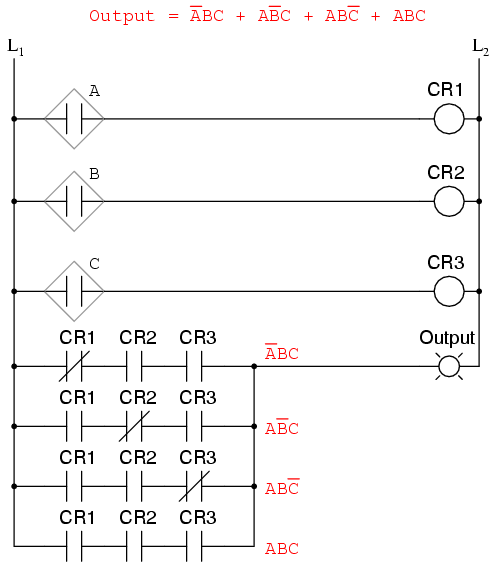Unfortunately, both of these circuits are quite complex, and could benefit from simplification. Using Boolean algebra techniques, the expression may be significantly simplified: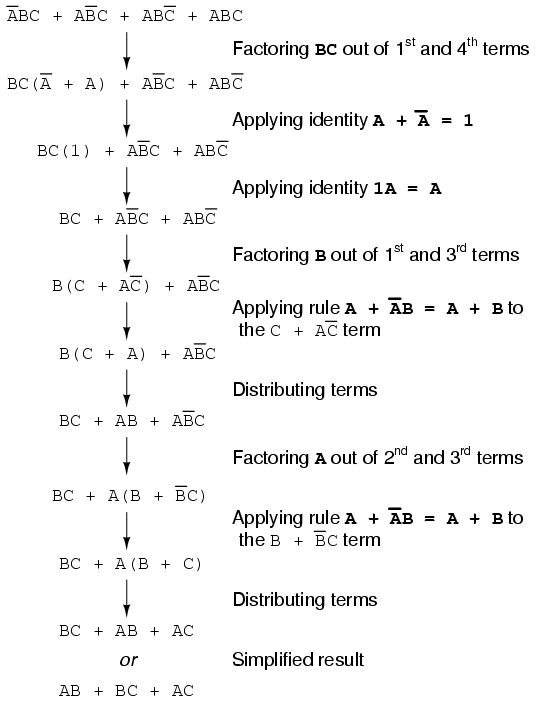As a result of the simplification, we can now build much simpler logic circuits performing the same function, in either gate or relay form: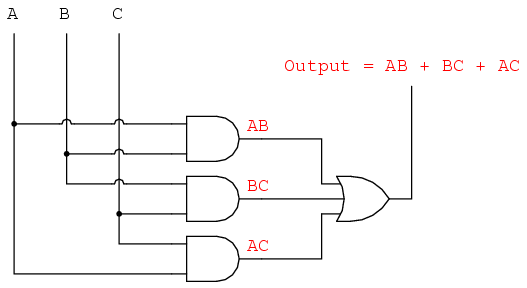Either one of these circuits will adequately perform the task of operating the incinerator waste valve based on a flame verification from two out of the three flame sensors. At minimum, this is what we need to have a safe incinerator system. We can, however, extend the functionality of the system by adding to it logic circuitry designed to detect if any one of the sensors does not agree with the other two.

If all three sensors are operating properly, they should detect flame with equal accuracy. Thus, they should either all register “low” (000: no flame) or all register “high” (111: good flame). Any other output combination (001, 010, 011, 100, 101, or 110) constitutes a disagreement between sensors, and may therefore serve as an indicator of a potential sensor failure. If we added circuitry to detect any one of the six “sensor disagreement” conditions, we could use the output of that circuitry to activate an alarm. Whoever is monitoring the incinerator would then exercise judgment in either continuing to operate with a possible failed sensor (inputs: 011, 101, or 110), or shut the incinerator down to be absolutely safe. Also, if the incinerator is shut down (no flame), and one or more of the sensors still indicates flame (001, 010, 011, 100, 101, or 110) while the other(s) indicate(s) no flame, it will be known that a definite sensor problem exists.

The first step in designing this “sensor disagreement” detection circuit is to write a truth table describing its behavior. Since we already have a truth table describing the output of the “good flame” logic circuit, we can simply add another output column to the table to represent the second circuit, and make a table representing the entire logic system: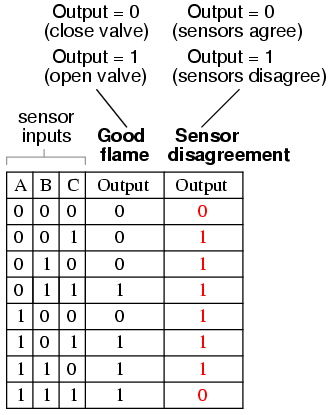While it is possible to generate a Sum-Of-Products expression for this new truth table column, it would require six terms, of three variables each! Such a Boolean expression would require many steps to simplify, with a large potential for making algebraic errors:An alternative to generating a Sum-Of-Products expression to account for all the “high” (1) output conditions in the truth table is to generate a Product-Of-Sums, or POS, expression, to account for all the “low” (0) output conditions instead. Being that there are much fewer instances of a “low” output in the last truth table column, the resulting Product-Of-Sums expression should contain fewer terms. As its name suggests, a Product-Of-Sums expression is a set of added terms (sums), which are multiplied (product) together. An example of a POS expression would be (A + B)(C + D), the product of the sums “A + B” and “C + D”.

To begin, we identify which rows in the last truth table column have “low” (0) outputs, and write a Boolean sum term that would equal 0 for that row’s input conditions. For instance, in the first row of the truth table, where A=0, B=0, and C=0, the sum term would be (A + B + C), since that term would have a value of 0 if and only if A=0, B=0, and C=0:Only one other row in the last truth table column has a “low” (0) output, so all we need is one more sum term to complete our Product-Of-Sums expression. This last sum term represents a 0 output for an input condition of A=1, B=1 and C=1. Therefore, the term must be written as (A’ + B’+ C’), because only the sum of the complemented input variables would equal 0 for that condition only: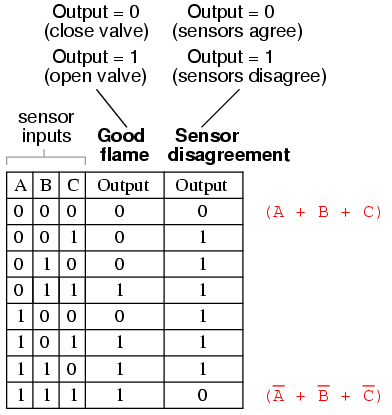The completed Product-Of-Sums expression, of course, is the multiplicative combination of these two sum terms: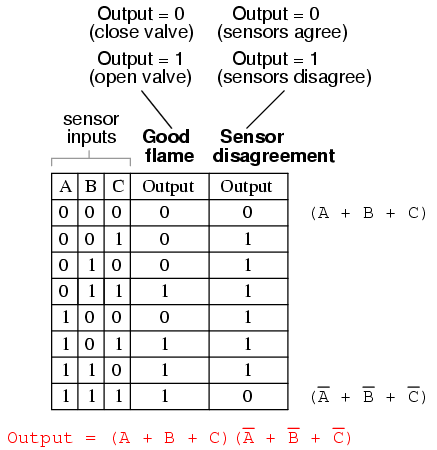Whereas a Sum-Of-Products expression could be implemented in the form of a set of AND gates with their outputs connecting to a single OR gate, a Product-Of-Sums expression can be implemented as a set of OR gates feeding into a single AND gate: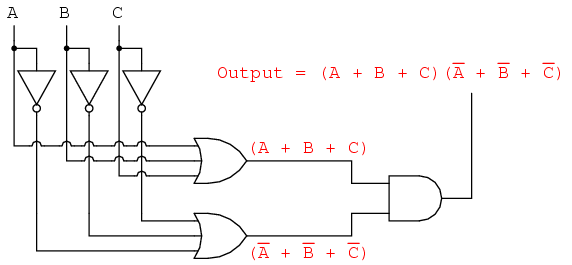Correspondingly, whereas a Sum-Of-Products expression could be implemented as a parallel collection of series-connected relay contacts, a Product-Of-Sums expression can be implemented as a series collection of parallel-connected relay contacts: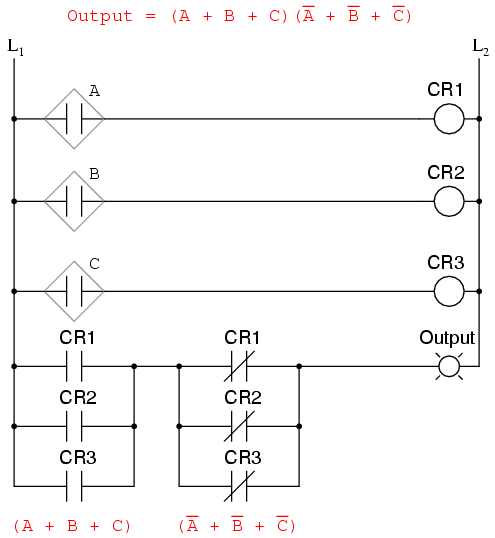The previous two circuits represent different versions of the “sensor disagreement” logic circuit only, not the “good flame” detection circuit(s). The entire logic system would be the combination of both “good flame” and “sensor disagreement” circuits, shown on the same diagram.

Implemented in a Programmable Logic Controller (PLC), the entire logic system might resemble something like this:As you can see, both the Sum-Of-Products and Products-Of-Sums standard Boolean forms are powerful tools when applied to truth tables. They allow us to derive a Boolean expression — and ultimately, an actual logic circuit — from nothing but a truth table, which is a written specification for what we want a logic circuit to do. To be able to go from a written specification to an actual circuit using simple, deterministic procedures means that it is possible to automate the design process for a digital circuit. In other words, a computer could be programmed to design a custom logic circuit from a truth table specification! The steps to take from a truth table to the final circuit are so unambiguous and direct that it requires little, if any, creativity or other original thought to execute them.

• REVIEW:
• Sum-Of-Products, or SOP, Boolean expressions may be generated from truth tables quite easily, by determining which rows of the table have an output of 1, writing one product term for each row, and finally summing all the product terms. This creates a Boolean expression representing the truth table as a whole.
• Sum-Of-Products expressions lend themselves well to implementation as a set of AND gates (products) feeding into a single OR gate (sum).
• Product-Of-Sums, or POS, Boolean expressions may also be generated from truth tables quite easily, by determining which rows of the table have an output of 0, writing one sum term for each row, and finally multiplyig all the sum terms. This creates a Boolean expression representing the truth table as a whole.
• Product-Of-Sums expressions lend themselves well to implementation as a set of OR gates (sums) feeding into a single AND gate (product).

Related

COMMENT songs